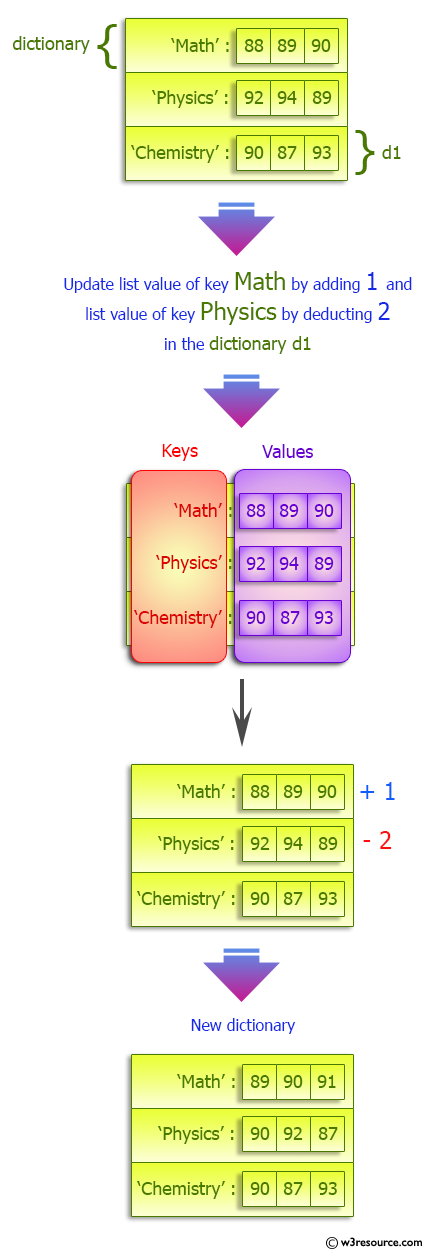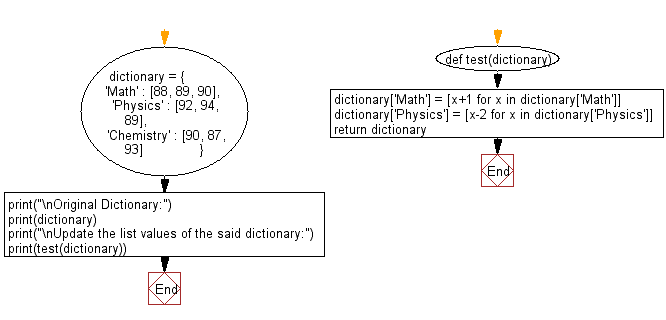﻿ Python: Update the list values within a given dictionary - w3resource# Python: Update the list values within a given dictionary

## Python dictionary: Exercise-51 with Solution

A Python Dictionary contains List as value. Write a Python program to update the list values in the said dictionary.

Sample Solution:

Python Code:

``````def test(dictionary):
dictionary['Math'] = [x+1 for x in dictionary['Math']]
dictionary['Physics'] = [x-2 for x in dictionary['Physics']]
return dictionary

dictionary = {
'Math' : [88, 89, 90],
'Physics' : [92, 94, 89],
'Chemistry' : [90, 87, 93]
}
print("\nOriginal Dictionary:")
print(dictionary)
print("\nUpdate the list values of the said dictionary:")
print(test(dictionary))
```
```

Sample Output:

```Original Dictionary:
{'Math': [88, 89, 90], 'Physics': [92, 94, 89], 'Chemistry': [90, 87, 93]}

Update the list values of the said dictionary:
{'Math': [89, 90, 91], 'Physics': [90, 92, 87], 'Chemistry': [90, 87, 93]}
```

Pictorial Presentation:Flowchart:## Visualize Python code execution:

The following tool visualize what the computer is doing step-by-step as it executes the said program:

Python Code Editor:

Have another way to solve this solution? Contribute your code (and comments) through Disqus.

What is the difficulty level of this exercise?

Test your Programming skills with w3resource's quiz.

﻿

## Python: Tips of the Day

Invokes the provided function after ms milliseconds:

Example:

```from time import sleep

def tips_delay(fn, ms, *args):
sleep(ms / 1000)
return fn(*args)
print(tips_delay(
lambda x: print(x),
1000,
'w3r'
))
```

Output:

```w3r
None
```